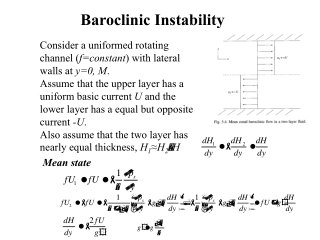DownloadDownload PresentationBaroclinic Instability

# Baroclinic Instability

Télécharger la présentation## Baroclinic Instability

- - - - - - - - - - - - - - - - - - - - - - - - - - - E N D - - - - - - - - - - - - - - - - - - - - - - - - - - -
##### Presentation Transcript

1. Baroclinic Instability Consider a uniformed rotating channel (f=constant) with lateral walls at y=0, M. Assume that the upper layer has a uniform basic current U and the lower layer has a equal but opposite current -U. Also assume that the two layer has nearly equal thickness, H1≈H2H Mean state

2. Stratification as a source of kinetic energy

3. Linearization of the potential vorticity equation For any given layer of thickness h=H(y)+ Where q is perturbation potential vorticity Mean potential vorticity gradient is

4. For our two layers, we have

5. (1) (2) (1)+(2): (1)-(2):

6. Assume the solutions have the form of Which satisfy the boundary condition of no normal flow at y=0, M

7. Long waves, are unstable and the growth rate is For l=k, the most unstable growth rate is at wavelength

8. If we take into account the  effect by noting that we have The frequency equation is The condition for instability is A necessary condition is

9. is a particular example of the more general necessary condition for instability that the mean vorticity gradient must change sign in the region In a two-layer model, if U > 0, then , the system is stable If also Therefore, the necessary condition for instability is so that

10. Kelvin-Helmholtz Instability The perturbation system is or are finite z=0,

11. Assume that we have The solutions satisfying the boundary conditions at Given We have

12. The unstable criterion is or with D the vertical (and horizontal) scale of perturbation Let we have Bulk Richardson number